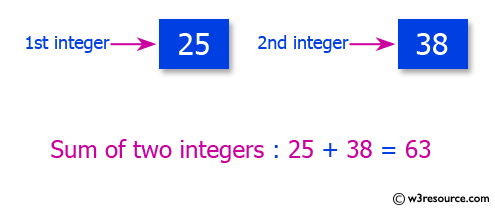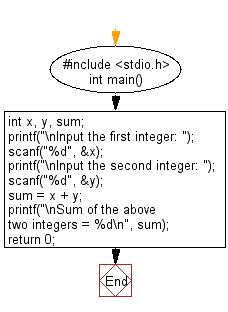﻿ C Program: Calculate the sum of the two integers - w3resource

# C Exercises: Calculate the sum of the two integers

## C Basic Declarations and Expressions: Exercise-9 with Solution

Write a C program that accepts two integers from the user and calculates the sum of the two integers.

Pictorial Presentation:C Code:

``````#include <stdio.h>
int main()
{
int x, y, sum; // Declare variables for two integers and their sum

// Prompt user for input and store in 'x'
printf("\nInput the first integer: ");
scanf("%d", &x);

// Prompt user for input and store in 'y'
printf("\nInput the second integer: ");
scanf("%d", &y);

sum = x + y; // Calculate the sum of 'x' and 'y'

// Print the sum
printf("\nSum of the above two integers = %d\n", sum);

return 0; // Indicate successful execution
}

``````

Sample Output:

```Input the first integer: 25

Input the second integer: 38

Sum of the above two integers = 63
```

Flowchart:C Programming Code Editor:

What is the difficulty level of this exercise?

Test your Programming skills with w3resource's quiz.

﻿